GFG App
Open AppBrowser
Continue

In this article, We are going to see seaborn color_palette(), which can be used for coloring the plot. Using the palette we can generate the point with different colors. In this below example we can see the palette can be responsible for generating the different colormap values.

Syntax: seaborn.color_palette(palette=None, n_colors=None, desat=None)

Parameters:

• palette: Name of palette or None to return current palette.
• n_colors: Number of colors in the palette.
• desat: Proportion to desaturate each color.

Returns: list of RGB tuples or matplotlib.colors.Colormap

We will classify the different ways for using color_palette() types −

• Qualitative
• Sequential
• Diverging

## Qualitative

A qualitative palette is used when the variable is categorical in nature, the color assigned to each group need to be distinct. Each possible value of the variable is assigned one color from a qualitative palette within a plot as shown in figure.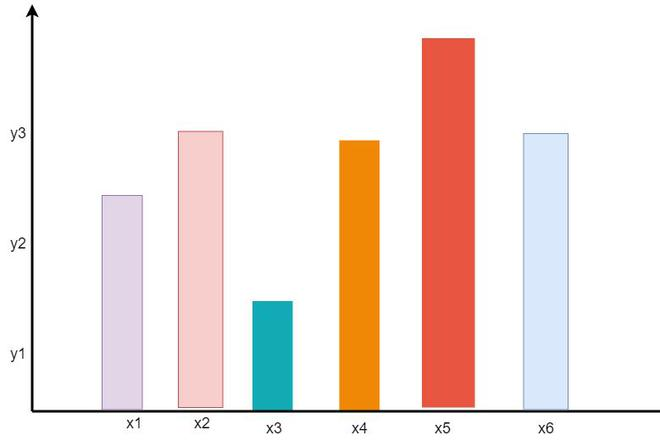Example:

## Python3

 `from` `matplotlib ``import` `pyplot as plt ` `import` `seaborn as sns ` `current_palette ``=` `sns.color_palette() ` `sns.palplot(current_palette) ` `plt.show()`

Output:## Sequential

In sequential palettes color moved sequentially from a lighter to a darker. When the variable assigned to be colored is numeric or has inherently ordered values, then it can be depicted with a sequential palette as shown in figure.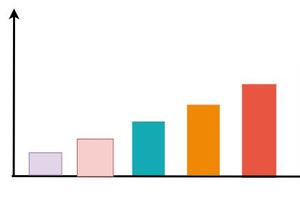Example:

## Python3

 `from` `matplotlib ``import` `pyplot as plt ` `import` `seaborn as sns ` `current_palette ``=` `sns.color_palette() ` `sns.palplot(sns.color_palette(``"Greys"``)) ` `plt.show()`

Output:## Diverging

When we work on mixed value like +ve and -ve(low and high values) then diverging palette is the best suit for visualization.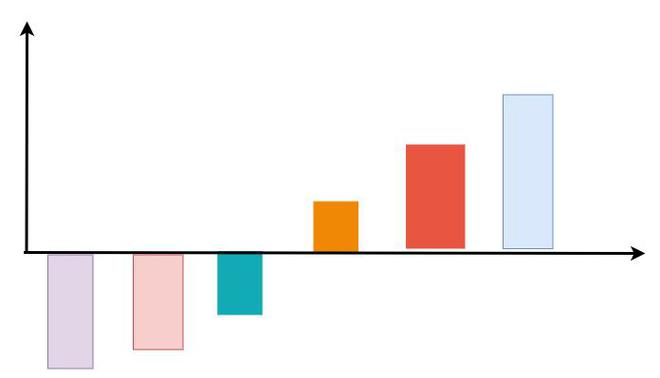Example:

## Python3

 `from` `matplotlib ``import` `pyplot as plt ` `import` `seaborn as sns ` `current_palette ``=` `sns.color_palette() ` `sns.palplot(sns.color_palette(``"terrain_r"``, ``7``)) ` `plt.show()`

Output:Let’s understand this with some examples:

Example 1:

In this example, we have used sns.color_palette() to construct a colormap and sns.palplot() to display the colors present in the colormap with “deep” attributes.

## Python3

 `# import module ` `import` `pandas as pd ` `import` `seaborn as sns ` ` `  `sns.palplot(sns.color_palette(``"deep"``, ``10``))`

Output:The possible value of the palette are:

‘Accent’, ‘Accent_r’, ‘Blues’, ‘Blues_r’, ‘BrBG’, ‘BrBG_r’, ‘BuGn’, ‘BuGn_r’, ‘BuPu’, ‘BuPu_r’, ‘CMRmap’, ‘CMRmap_r’, ‘Dark2’, ‘Dark2_r’, ‘GnBu’, ‘GnBu_r’,  ‘Greens’, ‘Greens_r’, ‘Greys’, ‘Greys_r’, ‘OrRd’, ‘OrRd_r’, ‘Oranges’, ‘Oranges_r’,  ‘PRGn’, ‘PRGn_r’, ‘Paired’, ‘Paired_r’, ‘Pastel1’, ‘Pastel1_r’, ‘Pastel2’,  ‘Pastel2_r’, ‘PiYG’, ‘PiYG_r’, ‘PuBu’, ‘PuBuGn’, ‘PuBuGn_r’, ‘PuBu_r’, ‘PuOr’, ‘PuOr_r’, ‘PuRd’, ‘PuRd_r’, ‘Purples’, ‘Purples_r’, ‘RdBu’, ‘RdBu_r’, ‘RdGy’, ‘RdGy_r’, ‘RdPu’, ‘RdPu_r’, ‘RdYlBu’, ‘RdYlBu_r’, ‘RdYlGn’, ‘RdYlGn_r’, ‘Reds’, ‘Reds_r’, ‘Set1’, ‘Set1_r’, ‘Set2’, ‘Set2_r’, ‘Set3’, ‘Set3_r’, ‘Spectral’, ‘Spectral_r’, ‘Wistia’, ‘Wistia_r’, ‘YlGn’, ‘YlGnBu’, ‘YlGnBu_r’, ‘YlGn_r’, ‘YlOrBr’, ‘YlOrBr_r’, ‘YlOrRd’, ‘YlOrRd_r’, ‘afmhot’, ‘afmhot_r’, ‘autumn’, ‘autumn_r’, ‘binary’, ‘binary_r’,  ‘bone’, ‘bone_r’, ‘brg’, ‘brg_r’, ‘bwr’, ‘bwr_r’, ‘cividis’, ‘cividis_r’, ‘cool’, ‘cool_r’,  ‘coolwarm’, ‘coolwarm_r’, ‘copper’, ‘copper_r’, ‘cubehelix’, ‘cubehelix_r’, ‘flag’, ‘flag_r’,  ‘gist_earth’, ‘gist_earth_r’, ‘gist_gray’, ‘gist_gray_r’, ‘gist_heat’, ‘gist_heat_r’, ‘gist_ncar’,  ‘gist_ncar_r’, ‘gist_rainbow’, ‘gist_rainbow_r’, ‘gist_stern’, ‘gist_stern_r’, ‘gist_yarg’,  ‘gist_yarg_r’, ‘gnuplot’, ‘gnuplot2’, ‘gnuplot2_r’, ‘gnuplot_r’, ‘gray’, ‘gray_r’, ‘hot’, ‘hot_r’,  ‘hsv’, ‘hsv_r’, ‘icefire’, ‘icefire_r’, ‘inferno’, ‘inferno_r’, ‘jet’, ‘jet_r’, ‘magma’, ‘magma_r’,  ‘mako’, ‘mako_r’, ‘nipy_spectral’, ‘nipy_spectral_r’, ‘ocean’, ‘ocean_r’, ‘pink’, ‘pink_r’,  ‘plasma’, ‘plasma_r’, ‘prism’, ‘prism_r’, ‘rainbow’, ‘rainbow_r’, ‘rocket’, ‘rocket_r’,  ‘seismic’, ‘seismic_r’, ‘spring’, ‘spring_r’, ‘summer’, ‘summer_r’, ‘tab10’, ‘tab10_r’,’tab20′, ‘tab20_r’, ‘tab20b’, ‘tab20b_r’, ‘tab20c’, ‘tab20c_r’, ‘terrain’, ‘terrain_r’, ‘turbo’,  ‘turbo_r’, ‘twilight’, ‘twilight_r’, ‘twilight_shifted’, ‘twilight_shifted_r’, ‘viridis’,  ‘viridis_r’, ‘vlag’, ‘vlag_r’, ‘winter’, ‘winter_r’

Example 2:

In this example, we have used sns.color_palette() to construct a colormap and sns.palplot() to display the colors present in the colormap with “muted” attributes.

## Python3

 `import` `pandas as pd ` `import` `seaborn as sns ` ` `  `sns.palplot(sns.color_palette(``"muted"``, ``10``)) `

Output:Example 3:

In this example, we have used sns.color_palette() to construct a colormap and sns.palplot() to display the colors present in the colormap with “bright” attributes.

## Python3

 `import` `pandas as pd ` `import` `seaborn as sns ` ` `  `sns.palplot(sns.color_palette(``"bright"``, ``10``)) `

Output: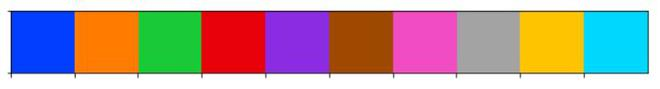Example 4:

In this example, we have used sns.color_palette() to construct a colormap and sns.palplot() to display the colors present in the colormap with “dark” attributes.

## Python3

 `import` `pandas as pd ` `import` `seaborn as sns ` ` `  `sns.palplot(sns.color_palette(``"dark"``, ``10``)) `

Output:Example 5:

In this example, we have used sns.color_palette() to construct a colormap and sns.palplot() to display the colors present in the colormap with “BuGn_r” attributes.

## Python3

 `import` `pandas as pd ` `import` `seaborn as sns ` ` `  `sns.palplot(sns.color_palette(``"BuGn_r"``, ``10``)) `

Output:Example 6:

In this example, creating an own color palette and set it as the current color palette

## Python3

 `import` `pandas as pd ` `import` `seaborn as sns ` ` `  `color ``=` `[``"green"``, ``"White"``, ``"Red"``, ``"Yellow"``, ``"Green"``, ``"Grey"``] ` `sns.set_palette(color) ` `sns.palplot(sns.color_palette())`

Output: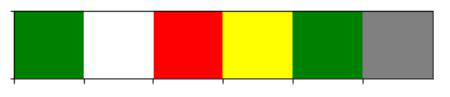My Personal Notes arrow_drop_up